Fluid Mechanics

# Bernoulli’s Equation

### Learning Objectives

By the end of this section, you will be able to:

• Explain the terms in Bernoulli’s equation
• Explain how Bernoulli’s equation is related to the conservation of energy
• Describe how to derive Bernoulli’s principle from Bernoulli’s equation
• Perform calculations using Bernoulli’s principle
• Describe some applications of Bernoulli’s principle

As we showed in (Figure), when a fluid flows into a narrower channel, its speed increases. That means its kinetic energy also increases. The increased kinetic energy comes from the net work done on the fluid to push it into the channel. Also, if the fluid changes vertical position, work is done on the fluid by the gravitational force.

A pressure difference occurs when the channel narrows. This pressure difference results in a net force on the fluid because the pressure times the area equals the force, and this net force does work. Recall the work-energy theorem,

${W}_{\text{net}}=\frac{1}{2}m{v}^{2}-\frac{1}{2}m{v}_{0}^{2}.$

The net work done increases the fluid’s kinetic energy. As a result, the pressure drops in a rapidly moving fluid whether or not the fluid is confined to a tube.

There are many common examples of pressure dropping in rapidly moving fluids. For instance, shower curtains have a disagreeable habit of bulging into the shower stall when the shower is on. The reason is that the high-velocity stream of water and air creates a region of lower pressure inside the shower, whereas the pressure on the other side remains at the standard atmospheric pressure. This pressure difference results in a net force, pushing the curtain inward. Similarly, when a car passes a truck on the highway, the two vehicles seem to pull toward each other. The reason is the same: The high velocity of the air between the car and the truck creates a region of lower pressure between the vehicles, and they are pushed together by greater pressure on the outside ((Figure)). This effect was observed as far back as the mid-1800s, when it was found that trains passing in opposite directions tipped precariously toward one another.

An overhead view of a car passing a truck on a highway. Air passing between the vehicles flows in a narrower channel and must increase its speed (${v}_{2}$ is greater than ${v}_{1}$), causing the pressure between them to drop (${p}_{\text{i}}$ is less than ${p}_{\text{o}}\right)\text{.}$ Greater pressure on the outside pushes the car and truck together.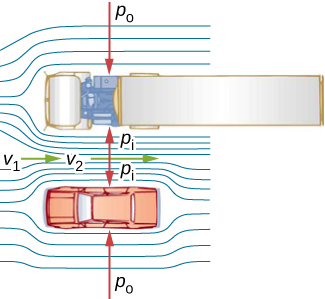### Energy Conservation and Bernoulli’s Equation

The application of the principle of conservation of energy to frictionless laminar flow leads to a very useful relation between pressure and flow speed in a fluid. This relation is called Bernoulli’s equation, named after Daniel Bernoulli (1700–1782), who published his studies on fluid motion in his book Hydrodynamica (1738).

Consider an incompressible fluid flowing through a pipe that has a varying diameter and height, as shown in (Figure). Subscripts 1 and 2 in the figure denote two locations along the pipe and illustrate the relationships between the areas of the cross sections A, the speed of flow v, the height from ground y, and the pressure p at each point. We assume here that the density at the two points is the same—therefore, density is denoted by $\rho$ without any subscripts—and since the fluid in incompressible, the shaded volumes must be equal.

The geometry used for the derivation of Bernoulli’s equation.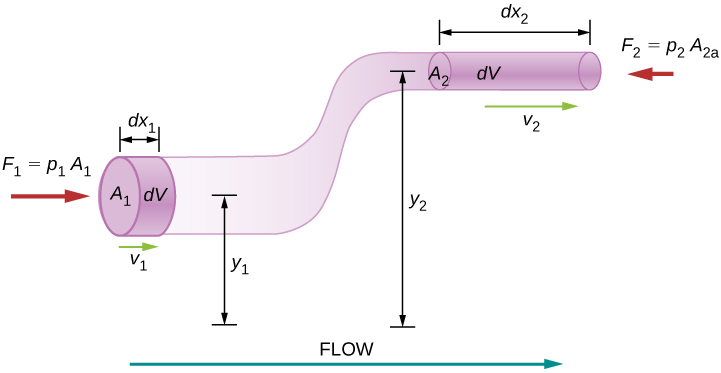We also assume that there are no viscous forces in the fluid, so the energy of any part of the fluid will be conserved. To derive Bernoulli’s equation, we first calculate the work that was done on the fluid:

$dW={F}_{1}d{x}_{1}-{F}_{2}d{x}_{2}$
$dW={p}_{1}{A}_{1}d{x}_{1}-{p}_{2}{A}_{2}d{x}_{2}={p}_{1}dV-{p}_{2}dV=\left({p}_{1}-{p}_{2}\right)dV.$

The work done was due to the conservative force of gravity and the change in the kinetic energy of the fluid. The change in the kinetic energy of the fluid is equal to

$dK=\frac{1}{2}{m}_{2}{v}_{2}^{2}-\frac{1}{2}{m}_{1}{v}_{1}^{2}=\frac{1}{2}\rho dV\left({v}_{2}^{2}-{v}_{1}^{2}\right).$

The change in potential energy is

$dU=mg{y}_{2}-mg{y}_{1}=\rho dVg\left({y}_{2}-{y}_{1}\right).$

The energy equation then becomes

$\begin{array}{ccc}\hfill dW& =\hfill & dK+dU\hfill \\ \hfill \left({p}_{1}-{p}_{2}\right)dV& =\hfill & \frac{1}{2}\rho dV\left({v}_{2}^{2}-{v}_{1}^{2}\right)+\rho dVg\left({y}_{2}-{y}_{1}\right)\hfill \\ \hfill \left({p}_{1}-{p}_{2}\right)& =\hfill & \frac{1}{2}\rho \left({v}_{2}^{2}-{v}_{1}^{2}\right)+\rho g\left({y}_{2}-{y}_{1}\right).\hfill \end{array}$

Rearranging the equation gives Bernoulli’s equation:

${p}_{1}+\frac{1}{2}\rho {v}_{1}^{2}+\rho g{y}_{1}={p}_{2}+\frac{1}{2}\rho {v}_{2}^{2}+\rho g{y}_{2}.$

This relation states that the mechanical energy of any part of the fluid changes as a result of the work done by the fluid external to that part, due to varying pressure along the way. Since the two points were chosen arbitrarily, we can write Bernoulli’s equation more generally as a conservation principle along the flow.

Bernoulli’s Equation

For an incompressible, frictionless fluid, the combination of pressure and the sum of kinetic and potential energy densities is constant not only over time, but also along a streamline:

$p+\frac{1}{2}\rho {v}^{2}+\rho gy=\text{constant}$

A special note must be made here of the fact that in a dynamic situation, the pressures at the same height in different parts of the fluid may be different if they have different speeds of flow.

### Analyzing Bernoulli’s Equation

According to Bernoulli’s equation, if we follow a small volume of fluid along its path, various quantities in the sum may change, but the total remains constant. Bernoulli’s equation is, in fact, just a convenient statement of conservation of energy for an incompressible fluid in the absence of friction.

The general form of Bernoulli’s equation has three terms in it, and it is broadly applicable. To understand it better, let us consider some specific situations that simplify and illustrate its use and meaning.

#### Bernoulli’s equation for static fluids

First consider the very simple situation where the fluid is static—that is, ${v}_{1}={v}_{2}=0.$ Bernoulli’s equation in that case is

${p}_{1}+\rho g{h}_{1}={p}_{2}+\rho g{h}_{2}.$

We can further simplify the equation by setting ${h}_{2}=0.$ (Any height can be chosen for a reference height of zero, as is often done for other situations involving gravitational force, making all other heights relative.) In this case, we get

${p}_{2}={p}_{1}+\rho g{h}_{1}.$

This equation tells us that, in static fluids, pressure increases with depth. As we go from point 1 to point 2 in the fluid, the depth increases by ${h}_{1}$, and consequently, ${p}_{2}$ is greater than ${p}_{1}$ by an amount $\rho g{h}_{1}$. In the very simplest case, ${p}_{1}$ is zero at the top of the fluid, and we get the familiar relationship $p=\rho gh$. $\left(\text{Recall that}\phantom{\rule{0.2em}{0ex}}p=\rho gh$ and $\text{Δ}{U}_{\text{g}}=\text{−}mgh.\right)$ Thus, Bernoulli’s equation confirms the fact that the pressure change due to the weight of a fluid is $\rho gh$. Although we introduce Bernoulli’s equation for fluid motion, it includes much of what we studied for static fluids earlier.

#### Bernoulli’s principle

Suppose a fluid is moving but its depth is constant—that is, ${h}_{1}={h}_{2}$. Under this condition, Bernoulli’s equation becomes

${p}_{1}+\frac{1}{2}\rho {v}_{1}^{2}={p}_{2}+\frac{1}{2}\rho {v}_{2}^{2}.$

Situations in which fluid flows at a constant depth are so common that this equation is often also called Bernoulli’s principle, which is simply Bernoulli’s equation for fluids at constant depth. (Note again that this applies to a small volume of fluid as we follow it along its path.) Bernoulli’s principle reinforces the fact that pressure drops as speed increases in a moving fluid: If ${v}_{2}$ is greater than ${v}_{1}$ in the equation, then ${p}_{2}$ must be less than ${p}_{1}$ for the equality to hold.

Calculating Pressure
In (Figure), we found that the speed of water in a hose increased from 1.96 m/s to 25.5 m/s going from the hose to the nozzle. Calculate the pressure in the hose, given that the absolute pressure in the nozzle is $1.01\phantom{\rule{0.2em}{0ex}}×\phantom{\rule{0.2em}{0ex}}{10}^{5}\phantom{\rule{0.2em}{0ex}}{\text{N/m}}^{2}$ (atmospheric, as it must be) and assuming level, frictionless flow.

Strategy
Level flow means constant depth, so Bernoulli’s principle applies. We use the subscript 1 for values in the hose and 2 for those in the nozzle. We are thus asked to find ${p1}_{}$.

Solution
Solving Bernoulli’s principle for ${p}_{1}$ yields

${p}_{1}={p}_{2}+\frac{1}{2}\rho {v}_{2}^{2}-\frac{1}{2}\rho {v}_{1}^{2}={p}_{2}+\frac{1}{2}\rho \left({v}_{2}^{2}-{v}_{1}^{2}\right).$

Substituting known values,

$\begin{array}{cc}\hfill {p}_{1}& =1.01\phantom{\rule{0.2em}{0ex}}×\phantom{\rule{0.2em}{0ex}}{10}^{5}\phantom{\rule{0.2em}{0ex}}{\text{N/m}}^{2}\text{+}\frac{1}{2}\left({10}^{3}\phantom{\rule{0.2em}{0ex}}{\text{kg/m}}^{3}\right)\left[{\text{(25.5 m/s)}}^{2}-{\text{(1.96 m/s)}}^{2}\right]\hfill \\ & =4.24\phantom{\rule{0.2em}{0ex}}×\phantom{\rule{0.2em}{0ex}}{10}^{5}\phantom{\rule{0.2em}{0ex}}{\text{N/m}}^{2}.\hfill \end{array}$

Significance
This absolute pressure in the hose is greater than in the nozzle, as expected, since v is greater in the nozzle. The pressure ${p}_{2}$ in the nozzle must be atmospheric, because the water emerges into the atmosphere without other changes in conditions.

### Applications of Bernoulli’s Principle

Many devices and situations occur in which fluid flows at a constant height and thus can be analyzed with Bernoulli’s principle.

#### Entrainment

People have long put the Bernoulli principle to work by using reduced pressure in high-velocity fluids to move things about. With a higher pressure on the outside, the high-velocity fluid forces other fluids into the stream. This process is called entrainment. Entrainment devices have been in use since ancient times as pumps to raise water to small heights, as is necessary for draining swamps, fields, or other low-lying areas. Some other devices that use the concept of entrainment are shown in (Figure).

Entrainment devices use increased fluid speed to create low pressures, which then entrain one fluid into another. (a) A Bunsen burner uses an adjustable gas nozzle, entraining air for proper combustion. (b) An atomizer uses a squeeze bulb to create a jet of air that entrains drops of perfume. Paint sprayers and carburetors use very similar techniques to move their respective liquids. (c) A common aspirator uses a high-speed stream of water to create a region of lower pressure. Aspirators may be used as suction pumps in dental and surgical situations or for draining a flooded basement or producing a reduced pressure in a vessel. (d) The chimney of a water heater is designed to entrain air into the pipe leading through the ceiling.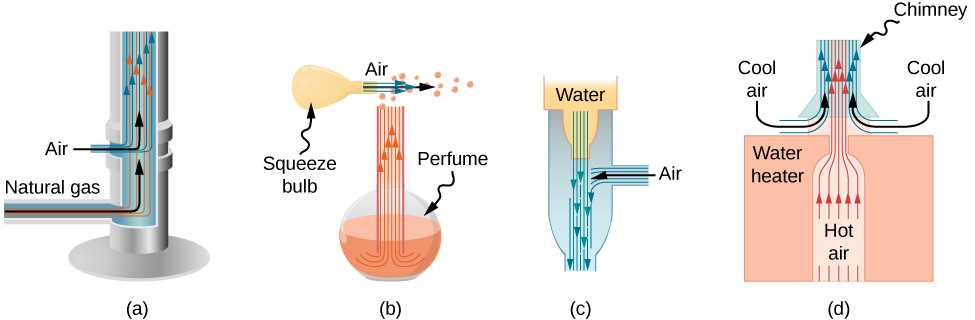#### Velocity measurement

(Figure) shows two devices that apply Bernoulli’s principle to measure fluid velocity. The manometer in part (a) is connected to two tubes that are small enough not to appreciably disturb the flow. The tube facing the oncoming fluid creates a dead spot having zero velocity (${v}_{1}=0$) in front of it, while fluid passing the other tube has velocity ${v}_{2}$. This means that Bernoulli’s principle as stated in

${p}_{1}+\frac{1}{2}\rho {v}_{1}^{2}={p}_{2}+\frac{1}{2}\rho {v}_{2}^{2}$

becomes

${p}_{1}={p}_{2}+\frac{1}{2}\rho {v}_{2}^{2}.$

Thus pressure ${p}_{2}$ over the second opening is reduced by $\frac{1}{2}\rho {v}_{2}^{2}$, so the fluid in the manometer rises by h on the side connected to the second opening, where

$h\propto \frac{1}{2}\rho {v}_{2}^{2}.$

(Recall that the symbol $\propto$ means “proportional to.”) Solving for ${v}_{2}$, we see that

${v}_{2}\propto \sqrt{h}.$

Part (b) shows a version of this device that is in common use for measuring various fluid velocities; such devices are frequently used as air-speed indicators in aircraft.

Measurement of fluid speed based on Bernoulli’s principle. (a) A manometer is connected to two tubes that are close together and small enough not to disturb the flow. Tube 1 is open at the end facing the flow. A dead spot having zero speed is created there. Tube 2 has an opening on the side, so the fluid has a speed v across the opening; thus, pressure there drops. The difference in pressure at the manometer is $\frac{1}{2}\rho {v}_{2}^{2}$, so h is proportional to $\frac{1}{2}\rho {v}_{2}^{2}.$ (b) This type of velocity measuring device is a Prandtl tube, also known as a pitot tube.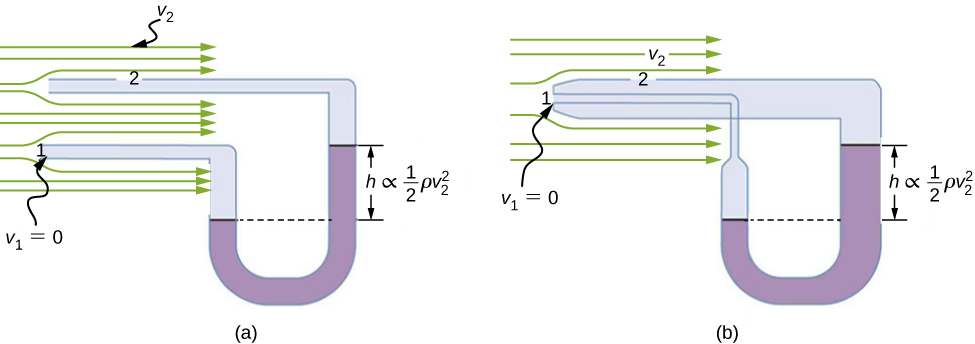#### A fire hose

All preceding applications of Bernoulli’s equation involved simplifying conditions, such as constant height or constant pressure. The next example is a more general application of Bernoulli’s equation in which pressure, velocity, and height all change.

Calculating Pressure: A Fire Hose Nozzle
Fire hoses used in major structural fires have an inside diameter of 6.40 cm ((Figure)). Suppose such a hose carries a flow of 40.0 L/s, starting at a gauge pressure of $1.62\phantom{\rule{0.2em}{0ex}}×\phantom{\rule{0.2em}{0ex}}{10}^{6}{\text{N/m}}^{2}$. The hose rises up 10.0 m along a ladder to a nozzle having an inside diameter of 3.00 cm. What is the pressure in the nozzle?

Pressure in the nozzle of this fire hose is less than at ground level for two reasons: The water has to go uphill to get to the nozzle, and speed increases in the nozzle. In spite of its lowered pressure, the water can exert a large force on anything it strikes by virtue of its kinetic energy. Pressure in the water stream becomes equal to atmospheric pressure once it emerges into the air.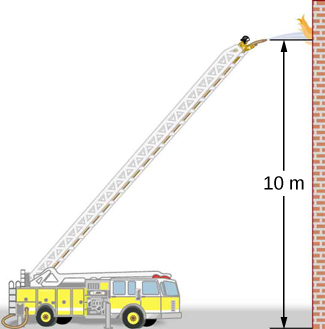Strategy
We must use Bernoulli’s equation to solve for the pressure, since depth is not constant.

Solution
Bernoulli’s equation is

${p}_{1}+\frac{1}{2}\rho {v}_{1}^{2}+\rho g{h}_{1}={p}_{2}+\frac{1}{2}\rho {v}_{2}^{2}+\rho g{h}_{2}$

where subscripts 1 and 2 refer to the initial conditions at ground level and the final conditions inside the nozzle, respectively. We must first find the speeds ${v}_{1}$ and ${v}_{2}$. Since $Q={A}_{1}{v}_{1}$, we get

${v}_{1}=\frac{Q}{{A}_{1}}=\frac{40.0\phantom{\rule{0.2em}{0ex}}×\phantom{\rule{0.2em}{0ex}}{10}^{-3}{\text{m}}^{3}\text{/}\text{s}}{\pi {\left(3.20\phantom{\rule{0.2em}{0ex}}×\phantom{\rule{0.2em}{0ex}}{10}^{-2}\text{m)}}^{2}}=12.4\text{m/s}.$

Similarly, we find

${v}_{2}=56.6\phantom{\rule{0.2em}{0ex}}\text{m/s}.$

This rather large speed is helpful in reaching the fire. Now, taking ${h}_{1}$ to be zero, we solve Bernoulli’s equation for ${p}_{2}$:

${p}_{2}={p}_{1}+\frac{1}{2}\rho \left({v}_{1}^{2}-{v}_{2}^{2}\right)-\rho g{h}_{2}.$

Substituting known values yields

$\begin{array}{cc}\hfill {p}_{2}& =1.62\phantom{\rule{0.2em}{0ex}}×\phantom{\rule{0.2em}{0ex}}{10}^{6}\phantom{\rule{0.2em}{0ex}}{\text{N/m}}^{2}+\frac{1}{2}\left(1000\phantom{\rule{0.2em}{0ex}}{\text{kg/m}}^{3}\right)\left[{\text{(12.4 m/s)}}^{2}-{\text{(56.6 m/s)}}^{2}\right]\hfill \\ & \phantom{\rule{0.1em}{0ex}}-\phantom{\rule{0.1em}{0ex}}\left(1000\phantom{\rule{0.2em}{0ex}}{\text{kg/m}}^{3}\right)\left(9.80\phantom{\rule{0.2em}{0ex}}{\text{m/s}}^{2}\right)\left(10.0\phantom{\rule{0.2em}{0ex}}\text{m}\right)\hfill \\ & =0.\hfill \end{array}$

Significance
This value is a gauge pressure, since the initial pressure was given as a gauge pressure. Thus, the nozzle pressure equals atmospheric pressure as it must, because the water exits into the atmosphere without changes in its conditions.

### Summary

• Bernoulli’s equation states that the sum on each side of the following equation is constant, or the same at any two points in an incompressible frictionless fluid:
${p}_{1}+\frac{1}{2}\rho {v}_{1}^{2}+\rho g{h}_{1}={p}_{2}+\frac{1}{2}\rho {v}_{2}^{2}+\rho g{h}_{2}.$
• Bernoulli’s principle is Bernoulli’s equation applied to situations in which the height of the fluid is constant. The terms involving depth (or height h) subtract out, yielding
${p}_{1}+\frac{1}{2}\rho {v}_{1}^{2}={p}_{2}+\frac{1}{2}\rho {v}_{2}^{2}.$
• Bernoulli’s principle has many applications, including entrainment and velocity measurement.

### Conceptual Questions

You can squirt water from a garden hose a considerably greater distance by partially covering the opening with your thumb. Explain how this works.

Water is shot nearly vertically upward in a decorative fountain and the stream is observed to broaden as it rises. Conversely, a stream of water falling straight down from a faucet narrows. Explain why.

The mass of water that enters a cross-sectional area must equal the amount that leaves. From the continuity equation, we know that the density times the area times the velocity must remain constant. Since the density of the water does not change, the velocity times the cross-sectional area entering a region must equal the cross-sectional area times the velocity leaving the region. Since the velocity of the fountain stream decreases as it rises due to gravity, the area must increase. Since the velocity of the faucet stream speeds up as it falls, the area must decrease.

Look back to (Figure). Answer the following two questions. Why is ${p}_{o}$ less than atmospheric? Why is ${p}_{o}$ greater than ${p}_{i}$?

A tube with a narrow segment designed to enhance entrainment is called a Venturi, such as shown below. Venturis are very commonly used in carburetors and aspirators. How does this structure bolster entrainment?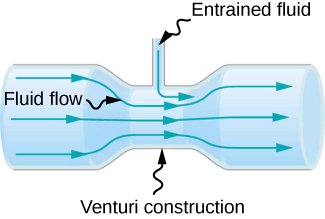When the tube narrows, the fluid is forced to speed up, thanks to the continuity equation and the work done on the fluid. Where the tube is narrow, the pressure decreases. This means that the entrained fluid will be pushed into the narrow area.

Some chimney pipes have a T-shape, with a crosspiece on top that helps draw up gases whenever there is even a slight breeze. Explain how this works in terms of Bernoulli’s principle.

Is there a limit to the height to which an entrainment device can raise a fluid? Explain your answer.

The work done by pressure can be used to increase the kinetic energy and to gain potential energy. As the height becomes larger, there is less energy left to give to kinetic energy. Eventually, there will be a maximum height that cannot be overcome.

Why is it preferable for airplanes to take off into the wind rather than with the wind?

Roofs are sometimes pushed off vertically during a tropical cyclone, and buildings sometimes explode outward when hit by a tornado. Use Bernoulli’s principle to explain these phenomena.

Because of the speed of the air outside the building, the pressure outside the house decreases. The greater pressure inside the building can essentially blow off the roof or cause the building to explode.

It is dangerous to stand close to railroad tracks when a rapidly moving commuter train passes. Explain why atmospheric pressure would push you toward the moving train.

Water pressure inside a hose nozzle can be less than atmospheric pressure due to the Bernoulli effect. Explain in terms of energy how the water can emerge from the nozzle against the opposing atmospheric pressure.

The air inside the hose has kinetic energy due to its motion. The kinetic energy can be used to do work against the pressure difference.

David rolled down the window on his car while driving on the freeway. An empty plastic bag on the floor promptly flew out the window. Explain why.

Based on Bernoulli’s equation, what are three forms of energy in a fluid? (Note that these forms are conservative, unlike heat transfer and other dissipative forms not included in Bernoulli’s equation.)

Potential energy due to position, kinetic energy due to velocity, and the work done by a pressure difference.

The old rubber boot shown below has two leaks. To what maximum height can the water squirt from Leak 1? How does the velocity of water emerging from Leak 2 differ from that of Leak 1? Explain your responses in terms of energy.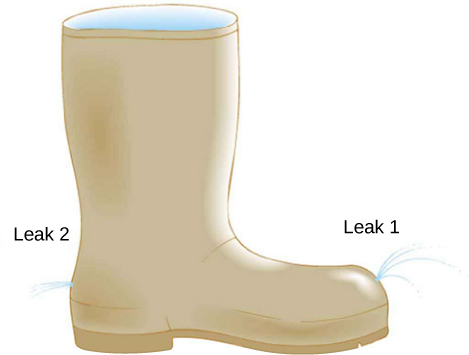Water pressure inside a hose nozzle can be less than atmospheric pressure due to the Bernoulli effect. Explain in terms of energy how the water can emerge from the nozzle against the opposing atmospheric pressure.

The water has kinetic energy due to its motion. This energy can be converted into work against the difference in pressure.

### Problems

Verify that pressure has units of energy per unit volume.

$\begin{array}{}\\ \\ \hfill F& =\hfill & pA⇒p=\frac{F}{A},\hfill \\ \hfill \left[p\right]& =\hfill & {\text{N/m}}^{2}=\text{N}·{\text{m/m}}^{3}={\text{J/m}}^{3}=\text{energy/volume}\hfill \end{array}$

Suppose you have a wind speed gauge like the pitot tube shown in (Figure). By what factor must wind speed increase to double the value of h in the manometer? Is this independent of the moving fluid and the fluid in the manometer?

If the pressure reading of your pitot tube is 15.0 mm Hg at a speed of 200 km/h, what will it be at 700 km/h at the same altitude?

−135 mm Hg

Every few years, winds in Boulder, Colorado, attain sustained speeds of 45.0 m/s (about 100 mph) when the jet stream descends during early spring. Approximately what is the force due to the Bernoulli equation on a roof having an area of $220{\text{m}}^{2}$? Typical air density in Boulder is $1.14{\text{kg/m}}^{3}$, and the corresponding atmospheric pressure is $8.89\phantom{\rule{0.2em}{0ex}}×\phantom{\rule{0.2em}{0ex}}{10}^{4}{\text{N/m}}^{2}$. (Bernoulli’s principle as stated in the text assumes laminar flow. Using the principle here produces only an approximate result, because there is significant turbulence.)

What is the pressure drop due to the Bernoulli Effect as water goes into a 3.00-cm-diameter nozzle from a 9.00-cm-diameter fire hose while carrying a flow of 40.0 L/s? (b) To what maximum height above the nozzle can this water rise? (The actual height will be significantly smaller due to air resistance.)

a. $1.58\phantom{\rule{0.2em}{0ex}}×\phantom{\rule{0.2em}{0ex}}{10}^{6}\phantom{\rule{0.2em}{0ex}}{\text{N/m}}^{2}$; b. 163 m

(a) Using Bernoulli’s equation, show that the measured fluid speed v for a pitot tube, like the one in (Figure)(b), is given by $v={\left(\frac{2{\rho }^{\prime }gh}{\rho }\right)}^{1\text{/}2}$, where h is the height of the manometer fluid, ${\rho }^{\prime }$ is the density of the manometer fluid, $\rho$ is the density of the moving fluid, and g is the acceleration due to gravity. (Note that v is indeed proportional to the square root of h, as stated in the text.) (b) Calculate v for moving air if a mercury manometer’s h is 0.200 m.

A container of water has a cross-sectional area of $A=0.1\phantom{\rule{0.2em}{0ex}}{\text{m}}^{2}$. A piston sits on top of the water (see the following figure). There is a spout located 0.15 m from the bottom of the tank, open to the atmosphere, and a stream of water exits the spout. The cross sectional area of the spout is ${A}_{\text{s}}=7.0\phantom{\rule{0.2em}{0ex}}×\phantom{\rule{0.2em}{0ex}}{10}^{-4}{\text{m}}^{2}$. (a) What is the velocity of the water as it leaves the spout? (b) If the opening of the spout is located 1.5 m above the ground, how far from the spout does the water hit the floor? Ignore all friction and dissipative forces.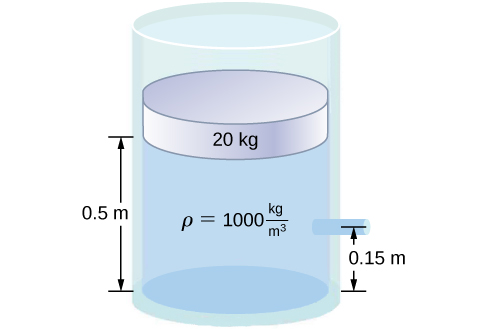a. ${v}_{2}=3.28\frac{\text{m}}{\text{s}}$;

b. $t=0.55\phantom{\rule{0.2em}{0ex}}\text{s}$

$x=vt=1.81\phantom{\rule{0.2em}{0ex}}\text{m}$

A fluid of a constant density flows through a reduction in a pipe. Find an equation for the change in pressure, in terms of ${v}_{1},{A}_{1},{A}_{2}$, and the density.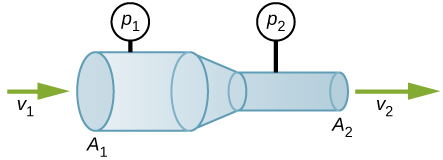### Glossary

Bernoulli’s equation
equation resulting from applying conservation of energy to an incompressible frictionless fluid: $p+\frac{1}{2}\rho {v}^{2}+\rho gh=\text{constant},$ throughout the fluid
Bernoulli’s principle
Bernoulli’s equation applied at constant depth:

${p}_{1}+\frac{1}{2}\rho {v}_{1}^{2}={p}_{2}+\frac{1}{2}\rho {v}_{2}^{2}$